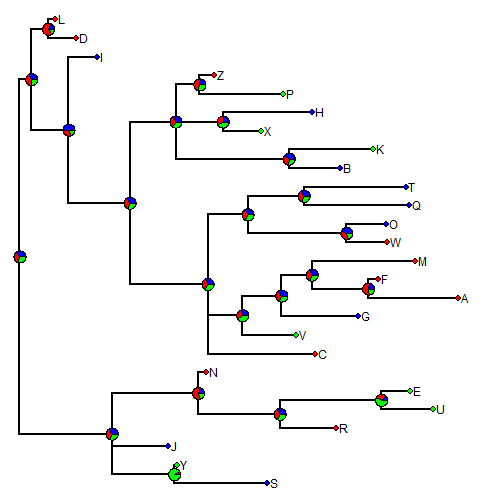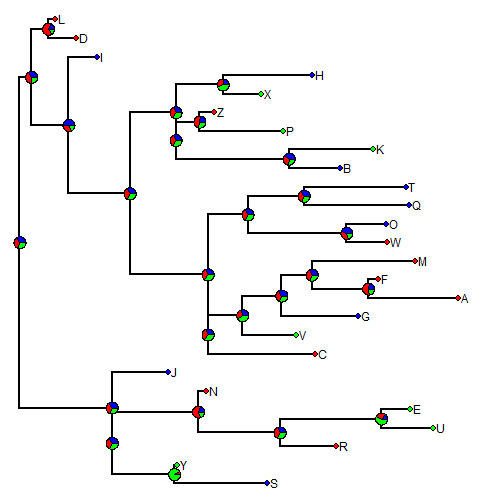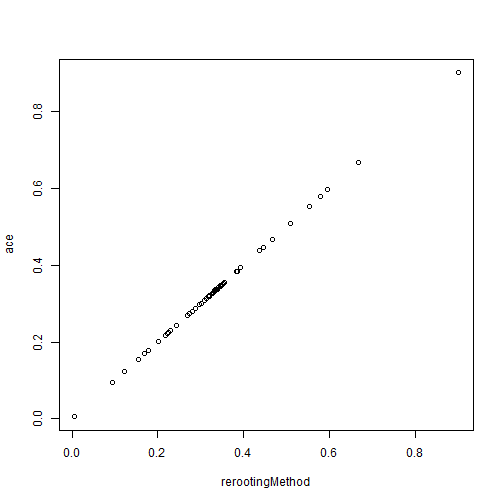## Friday, September 18, 2015

### Imperceptible update to rerootingMethod for ancestral state reconstruction

I just made an update to the phytools function `rerootingMethod` which does marginal ancestral state estimation using the 'rerooting method' of Yang (1996). This method is essentially redundant (now) with `ace(...,type="discrete")` under its default settings, although it does not permit assymetric models of trait evolution (i.e., models in which the backward & forward rates for a particular transition type are allowed to assume different values). The only advantage of this method is that the function does permit polytomies. Prior versions also permitted polytomies; however this was by resolving polytomies internally, estimating ancestral states, and then matching the nodes between the fully resolved tree and its original, multifurcating counterpart. Now that `rerootingMethod` uses my new Mk model fitting function internally (`fitMk`), this is no longer necessary.

We can see this as follows:

``````library(devtools)
install_github("liamrevell/phytools",quiet=TRUE)
library(phytools)
``````
``````## Loading required package: ape
``````
``````set.seed(1)
## simulate tree with polytomies & data
tree<-rtree(n=26,tip.label=LETTERS)
tree\$edge.length[which(tree\$edge[,2]==47)]<-0
tree\$edge.length[which(tree\$edge[,2]==38)]<-0
tree\$edge.length[which(tree\$edge[,2]==29)]<-0
tree<-di2multi(tree)
plotTree(tree)
Q<-matrix(c(-1,1,0,1,-2,1,0,1,-1),3,3)
rownames(Q)<-colnames(Q)<-letters[1:3]
x<-sim.history(tree,Q)\$states
``````
``````## Done simulation(s).
``````
``````x
``````
``````##   S   Y   J   R   U   E   N   C   V   G   A   F   M   W   O   Q   T   B
## "a" "c" "a" "b" "c" "c" "b" "b" "c" "a" "b" "b" "b" "b" "a" "a" "a" "a"
##   K   X   H   P   Z   I   D   L
## "c" "c" "a" "c" "b" "a" "b" "b"
``````
``````## fit model & estimate ancestral states using rerootingMethod
model<-matrix(c(0,1,0,1,0,1,0,1,0),3,3)
rownames(model)<-colnames(model)<-letters[1:3]
fit1<-rerootingMethod(tree,x,model=model)
plotTree(tree)
nodelabels(pie=fit1\$marginal.anc,piecol=setNames(c("blue","red","green"),
c("a","b","c")),cex=0.6)
tiplabels(pie=to.matrix(x[tree\$tip.label],c("a","b","c")),
piecol=setNames(c("blue","red","green"),c("a","b","c")),cex=0.3)
````````````## compare to ace
fit2<-ace(x,tree,type="discrete",model=model) ## doesn't work
``````
``````## Error in ace(x, tree, type = "discrete", model = model): "phy" is not rooted AND fully dichotomous.
``````
``````fit3<-ace(x,multi2di(tree),type="discrete",model=model) ## doesn't work
``````
``````## Error in ace(x, multi2di(tree), type = "discrete", model = model): some branches have length zero or negative
``````
``````tt<-multi2di(tree)
tt\$edge.length[tt\$edge.length==0]<-1e-8
fit4<-ace(x,tt,type="discrete",model=model) ## works
## compare to rerootingMethod
M<-matchNodes(tree,tt) ## first match nodes between the trees
plotTree(tt)
nodelabels(pie=fit4\$lik.anc,piecol=setNames(c("blue","red","green"),
c("a","b","c")),cex=0.6)
tiplabels(pie=to.matrix(x[tt\$tip.label],c("a","b","c")),
piecol=setNames(c("blue","red","green"),c("a","b","c")),cex=0.3)
````````````plot(fit1\$marginal.anc,fit4\$lik.anc[M[,2]-Ntip(tt),],
xlab="rerootingMethod",ylab="ace")
``````That's it.